# 【Python数据挖掘课程】五.线性回归知识及预测糖尿病实例

今天主要讲述的内容是关于一元线性回归的知识，Python实现，包括以下内容：
1.机器学习常用数据集介绍
2.什么是线性回顾
3.LinearRegression使用方法

希望这篇文章对你有所帮助，尤其是刚刚接触数据挖掘以及大数据的同学，同时准备尝试以案例为主的方式进行讲解。如果文章中存在不足或错误的地方，还请海涵~
同时这篇文章是我上课的内容，所以参考了一些知识，强烈推荐大家学习斯坦福的机器学习Ng教授课程和Scikit-Learn中的内容。由于自己数学不是很好，自己也还在学习中，所以文章以代码和一元线性回归为主，数学方面的当自己学到一定的程度，才能进行深入的分享及介绍。抱歉~

## 一. 数据集介绍

1.diabetes dataset数据集
数据集参考：http://scikit-learn.org/stable/datasets/
这是一个糖尿病的数据集，主要包括442行数据，10个属性值，分别是：Age(年龄)、性别(Sex)、Body mass index(体质指数)、Average Blood Pressure(平均血压)、S1~S6一年后疾病级数指标。Target为一年后患疾病的定量指标。输出如下所示：

# -*- coding: utf-8 -*-
"""
Created on Thu Oct 27 02:37:05 2016

@author: yxz15
"""

from sklearn import datasets
print diabetes.data                                         #数据
print diabetes.target                                       #类标
print u'总行数: ', len(diabetes.data), len(diabetes.target) #数据总行数
print u'特征数: ', len(diabetes.data)                    #每行数据集维数
print u'数据类型: ', diabetes.data.shape                    #类型
print type(diabetes.data), type(diabetes.target)            #数据集类型

"""
[[ 0.03807591  0.05068012  0.06169621 ..., -0.00259226  0.01990842
-0.01764613]
[-0.00188202 -0.04464164 -0.05147406 ..., -0.03949338 -0.06832974
-0.09220405]
...
[-0.04547248 -0.04464164 -0.0730303  ..., -0.03949338 -0.00421986
0.00306441]]

[ 151.   75.  141.  206.  135.   97.  138.   63.  110.  310.  101.
...
64.   48.  178.  104.  132.  220.   57.]

<type 'numpy.ndarray'> <type 'numpy.ndarray'>
"""

2.sklearn常见数据集
常见的sklearn数据集包括，强烈推荐下面这篇文章：
sklearn包含一些不许要下载的toy数据集，见下表，包括波士顿房屋数据集、鸢尾花数据集、糖尿病数据集、手写字数据集和健身数据集等。3.UCI数据集
常用数据集包括：http://archive.ics.uci.edu/ml/datasets.html## 二. 什么是线性回归

1.机器学习简述
机器学习（Machine Learning ）包括：
a.监督学习（Supervised Learning）：回归（Regression）、分类(Classification)
例：训练过程中知道结果。小孩水果分类，给他苹果告诉他是苹果，反复训练学习。在给他说过，问他是什么？他回答准确，如果是桃子，他不能回答为苹果。

b.无监督学习（Unsupervised Learning）：聚类（Clustering）
例：训练过程中不知道结果。给小孩一堆水果，如苹果、橘子、桃子，小孩开始不知道需要分类的水果是什么，让小孩对水果进行分类。分类完成后，给他一个苹果，小孩应该把它放到苹果堆中。

c.增强学习（Reinforcement Learning）
例：ML过程中，对行为做出评价，评价有正面的和负面两种。通过学习评价，程序应做出更好评价的行为。

d.推荐系统（Recommender System）

2.斯坦福公开课：第二课 单变量线性回归
这是NG教授的很著名的课程，这里主要引用52nlp的文章，真的太完美了。推荐阅读该作者的更多文章：
Coursera公开课笔记: 斯坦福大学机器学习第二课"单变量线性回归(Linear regression with one variable)"

<1>模型表示（Model Representation）
房屋价格预测问题，有监督学习问题。每个样本的输入都有正确输出或答案，它也是一个回归问题，预测一个真实值的输出。
训练集表示如下：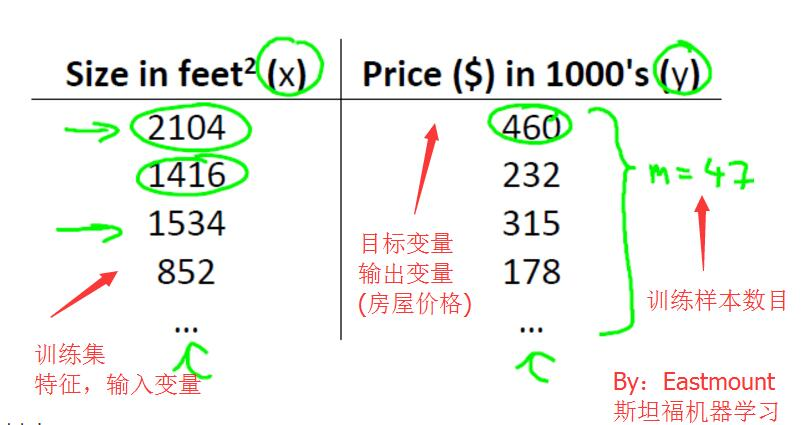对于房价预测问题，讯息过程如下所示：其中x代表房屋的大小，y代表预测的价格，h（hypothesis）将输入变量映射到输出变量y中，如何表示h呢？ 可以表示如下公式，简写为h(x)，即带一个变量的线性回归或单变量线性回归问题。<2>成本函数（Cost Function）
对于上面的公式函数h(x)，如何求theta0和theta1参数呢？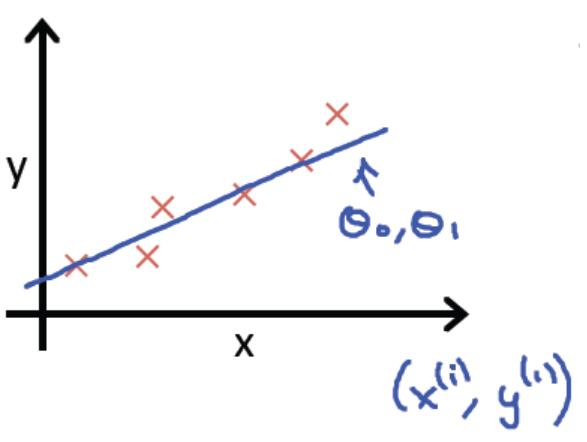构想： 对于训练集(x, y)，选取参数 θ0 θ1 使得 hθ(x) 尽可能的接近y。如何做呢？一种做法就是求训练集的平方误差函数（squared error function）。
Cost Function可表示为：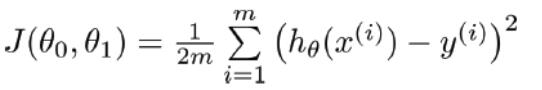并且选取合适的参数使其最小化，数学表示如下：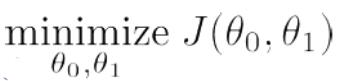总的来说，线性回归主要包括一下四个部分，分别是Hypothesis、Parameters、Cost Function、Goal。右图位简化版，theta0赋值为0。然后令 θ1 分别取1、0.5、-0.5等值，同步对比 hθ(x) J(θ0,θ1) 在二维坐标系中的变化情况，具体可参考原PPT中的对比图，很直观。

应用的场景之一最小值问题：

对于一些函数，例如 J(θ0,θ1)

目标:   minθ0,θ1J(θ0,θ1)

方法的框架:

a. 给 θ0 θ1 一个初始值，例如都等于0;

b. 每次改变 θ0 θ1 的时候都保持 J(θ0,θ1) 递减，直到达到一个我们满意的最小值；

对于任一 J(θ0,θ1)  , 初始位置不同，最终达到的极小值点也不同，例如以下例子：3.一元回归模型
转自文章：http://blog.sina.com.cn/s/blog_68c81f3901019hhp.html

<1>什么是线性回归？
回归函数的具体解释和定义，可查看任何一本“概率论与数理统计”的书。我看的是“陈希孺”的。
这里我讲几点：
１）统计回归分析的任务，就在于根据 x1,x2,...,xp 线性回归和Y的观察值，去估计函数f，寻求变量之间近似的函数关系。
２）我们常用的是，假定f函数的数学形式已知，其中若干个参数未知，要通过自变量和因变量的观察值去估计未知的参数值。这叫“参数回归”。其中应用最广泛的是f为线性函数的假设：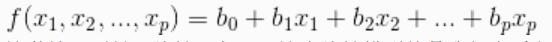这种情况叫做“线性回归”。
３）自变量只有一个时，叫做一元线性回归。
f(x) = b0+b1x
自变量有多个时，叫做多元线性回归。
f(x1,x2,...,xp) = b0 + b1x1 + b2x2 + ... + bpxp
４）分类(Classification)与回归(Regression)都属于监督学习，他们的区别在于：
分类：用于预测有限的离散值，如是否得了癌症（０，１），或手写数字的判断，是0,1,2,3,4,5,6,7,8还是9等。分类中，预测的可能的结果是有限的，且提前给定的。
回归：用于预测实数值，如给定了房子的面积，地段，和房间数，预测房子的价格。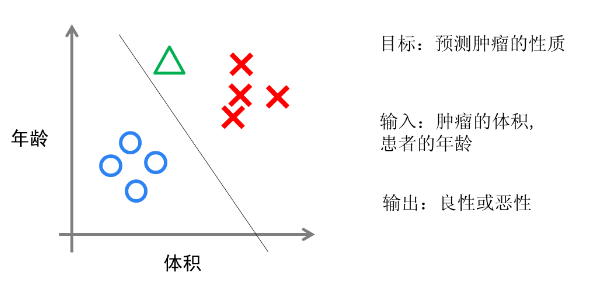<2>一元线性回归
假设：我们要预测房价。当前自变量(输入特征)是房子面积x，因变量是房价y.给定了一批训练集数据。 我们要做的是利用手上的训练集数据，得出x与y之间的函数f关系，并用f函数来预测任意面积x对应的房价。
假设x与y是线性关系，则我们可以接着假设一元线性回归函数如下来代表y的预测值：我们有训练集了，那么问题就成了如何利用现有的训练集来判定未知参数 (θ0,θ1) 的值，使其让h的值更接近实际值y? 训练集指的是已知x,y值的数据集合！

一种方法是计算它的成本函数(Cost function)，即预测出来的h的值与实际值y之间的方差的大小来决定当前的(θ0,θ1)值是否是最优的！
常用的成本函数是最小二乘法：<3>模型总结
整个一元线性回归通过下面这张图总结即可：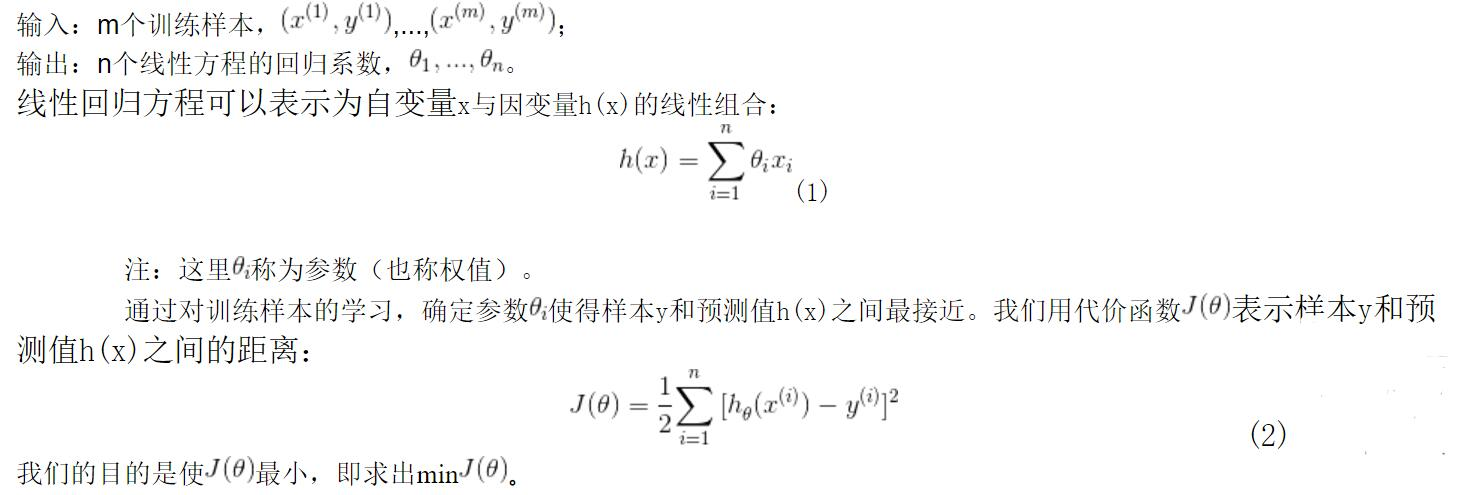最后，梯度下降和多元回归模型将继续学习，当我学到一定程度，再进行分享。
http://www.52nlp.cn/coursera公开课笔记-斯坦福大学机器学习第四课多变量

## 三. LinearRegression使用方法

LinearRegression模型在Sklearn.linear_model下，它主要是通过fit(x,y)的方法来训练模型，其中x为数据的属性，y为所属类型。
sklearn中引用回归模型的代码如下：

from sklearn import linear_model       #导入线性模型
regr = linear_model.LinearRegression() #使用线性回归
print regr


输出的函数原型如下所示：

LinearRegression(copy_X=True,
fit_intercept=True,
n_jobs=1,
normalize=False)
fit(x, y): 训练。分析模型参数，填充数据集。其中x为特征，y位标记或类属性。
predict(): 预测。它通过fit()算出的模型参数构成的模型，对解释变量进行预测其类属性。预测方法将返回预测值y_pred。

这里推荐"搬砖小工053"大神的文章，非常不错，强烈推荐。
引用他文章的例子，参考：scikit-learn : 线性回归，多元回归，多项式回归

# -*- coding: utf-8 -*-
"""
Created on Fri Oct 28 00:44:55 2016

@author: yxz15
"""

from sklearn import linear_model       #导入线性模型
import matplotlib.pyplot as plt        #绘图
import numpy as np

#X表示匹萨尺寸 Y表示匹萨价格
X = [, , , , ]
Y = [, , , [17.5], ]

print u'数据集X: ', X
print u'数据集Y: ', Y

#回归训练
clf = linear_model.LinearRegression() #使用线性回归
clf.fit(X, Y)                         #导入数据集
res = clf.predict(np.array().reshape(-1, 1)) #预测结果
print(u'预测一张12英寸匹萨价格：$%.2f' % res) #预测结果 X2 = [, , , ] Y2 = clf.predict(X2) #绘制线性回归图形 plt.figure() plt.title(u'diameter-cost curver') #标题 plt.xlabel(u'diameter') #x轴坐标 plt.ylabel(u'cost') #y轴坐标 plt.axis([0, 25, 0, 25]) #区间 plt.grid(True) #显示网格 plt.plot(X, Y, 'k.') #绘制训练数据集散点图 plt.plot(X2, Y2, 'g-') #绘制预测数据集直线 plt.show()  运行结果如下所示，首先输出数据集，同时调用sklearn包中的LinearRegression()回归函数，fit(X, Y)载入数据集进行训练，然后通过predict()预测数据12尺寸的匹萨价格，最后定义X2数组，预测它的价格。 数据集X: [, , , , ] 数据集Y: [, , , [17.5], ] 预测一张12英寸匹萨价格：$13.68
输出的图形如下所示：线性模型的回归系数W会保存在他的coef_方法中，截距保存在intercept_中。score(X,y,sample_weight=None) 评分函数，返回一个小于1的得分，可能会小于0。

print u'系数', clf.coef_
print u'截距', clf.intercept_
print u'评分函数', clf.score(X, Y)
'''

'''
其中具体的系数介绍推荐如下资料： sklearn学习笔记之简单线性回归 - Magle

## 四. 线性回归判断糖尿病

1.Diabetes数据集（糖尿病数据集）
糖尿病数据集包含442个患者的10个生理特征（年龄，性别、体重、血压）和一年以后疾病级数指标。
然后载入数据，同时将diabetes糖尿病数据集分为测试数据和训练数据，其中测试数据为最后20行，训练数据从0到-20行（不包含最后20行），即diabetes.data[:-20]。

from sklearn import datasets

#数据集

diabetes_x = diabetes.data[:, np.newaxis]  #获取一个特征
diabetes_x_temp = diabetes_x[:, :, 2]

diabetes_x_train = diabetes_x_temp[:-20]   #训练样本
diabetes_x_test = diabetes_x_temp[-20:]    #测试样本 后20行
diabetes_y_train = diabetes.target[:-20]   #训练标记
diabetes_y_test = diabetes.target[-20:]    #预测对比标记

print u'划分行数:', len(diabetes_x_temp), len(diabetes_x_train), len(diabetes_x_test)
print diabetes_x_test
输出结果如下所示，可以看到442个数据划分为422行进行训练回归模型，20行数据用于预测。输出的diabetes_x_test共20行数据，每行仅一个特征。
划分行数: 442 422 20
[[ 0.07786339]
[-0.03961813]
[ 0.01103904]
[-0.04069594]
[-0.03422907]
[ 0.00564998]
[ 0.08864151]
[-0.03315126]
[-0.05686312]
[-0.03099563]
[ 0.05522933]
[-0.06009656]
[ 0.00133873]
[-0.02345095]
[-0.07410811]
[ 0.01966154]
[-0.01590626]
[-0.01590626]
[ 0.03906215]
[-0.0730303 ]]

2.完整代码
改代码的任务是从生理特征预测疾病级数，但仅获取了一维特征，即一元线性回归。【线性回归】的最简单形式给数据集拟合一个线性模型，主要是通过调整一系列的参以使得模型的残差平方和尽量小。
线性模型：y = βX+b
X:数据 y：目标变量 β：回归系数 b:观测噪声（bias，偏差）
参考文章：Linear Regression Example - Scikit-Learn

# -*- coding: utf-8 -*-
"""
Created on Fri Oct 28 01:21:30 2016

@author: yxz15
"""

from sklearn import datasets
import matplotlib.pyplot as plt
import numpy as np

#数据集

#获取一个特征
diabetes_x_temp = diabetes.data[:, np.newaxis, 2]

diabetes_x_train = diabetes_x_temp[:-20]   #训练样本
diabetes_x_test = diabetes_x_temp[-20:]    #测试样本 后20行
diabetes_y_train = diabetes.target[:-20]   #训练标记
diabetes_y_test = diabetes.target[-20:]    #预测对比标记

#回归训练及预测
clf = linear_model.LinearRegression()
clf.fit(diabetes_x_train, diabetes_y_train)  #注: 训练数据集

#系数 残差平法和 方差得分
print 'Coefficients :\n', clf.coef_
print ("Residual sum of square: %.2f" %np.mean((clf.predict(diabetes_x_test) - diabetes_y_test) ** 2))
print ("variance score: %.2f" % clf.score(diabetes_x_test, diabetes_y_test))

#绘图
plt.title(u'LinearRegression Diabetes')   #标题
plt.xlabel(u'Attributes')                 #x轴坐标
plt.ylabel(u'Measure of disease')         #y轴坐标
#点的准确位置
plt.scatter(diabetes_x_test, diabetes_y_test, color = 'black')
#预测结果 直线表示
plt.plot(diabetes_x_test, clf.predict(diabetes_x_test), color='blue', linewidth = 3)
plt.show()

运行结果如下所示，包括系数、残差平方和、方差分数。

Coefficients :[ 938.23786125]
Residual sum of square: 2548.07
variance score: 0.47
绘制图形如下所示，每个点表示真实的值，而直线表示预测的结果，比较接近吧。同时绘制图形时，想去掉坐标具体的值，可增加如下代码：

plt.xticks(())
plt.yticks(())

## 五. 优化代码

下面是优化后的代码，增加了斜率、 截距的计算，同时增加了点图到线性方程的距离，保存图片设置像素。

# -*- coding: utf-8 -*-
"""
Created on Thu Dec 29 12:47:58 2011

"""
#第一步 数据集划分
from sklearn import datasets
import numpy as np

#获取数据 10*442
x = d.data
print u'获取x特征'
print len(x), x.shape
print x[:4]

#获取一个特征 第3列数据
x_one = x[:,np.newaxis, 2]
print x_one[:4]

#获取的正确结果
y = d.target
print u'获取的结果'
print y[:4]

#x特征划分
x_train = x_one[:-42]
x_test = x_one[-42:]
print len(x_train), len(x_test)
y_train = y[:-42]
y_test = y[-42:]
print len(y_train), len(y_test)

#第二步 线性回归实现
from sklearn import linear_model
clf = linear_model.LinearRegression()
print clf
clf.fit(x_train, y_train)
pre = clf.predict(x_test)
print u'预测结果'
print pre
print u'真实结果'
print y_test

#第三步 评价结果
cost = np.mean(y_test-pre)**2
print u'次方', 2**5
print u'平方和计算:', cost
print u'系数', clf.coef_
print u'截距', clf.intercept_
print u'方差', clf.score(x_test, y_test)

#第四步 绘图
import matplotlib.pyplot as plt
plt.title("diabetes")
plt.xlabel("x")
plt.ylabel("y")
plt.plot(x_test, y_test, 'k.')
plt.plot(x_test, pre, 'g-')

for idx, m in enumerate(x_test):
plt.plot([m, m],[y_test[idx],
pre[idx]], 'r-')

plt.savefig('power.png', dpi=300)

plt.show()


运行结果如下所示：
获取x特征
442 (442L, 10L)
[[ 0.03807591  0.05068012  0.06169621  0.02187235 -0.0442235  -0.03482076
-0.04340085 -0.00259226  0.01990842 -0.01764613]
[-0.00188202 -0.04464164 -0.05147406 -0.02632783 -0.00844872 -0.01916334
0.07441156 -0.03949338 -0.06832974 -0.09220405]
[ 0.08529891  0.05068012  0.04445121 -0.00567061 -0.04559945 -0.03419447
-0.03235593 -0.00259226  0.00286377 -0.02593034]
[-0.08906294 -0.04464164 -0.01159501 -0.03665645  0.01219057  0.02499059
-0.03603757  0.03430886  0.02269202 -0.00936191]]
[[ 0.06169621]
[-0.05147406]
[ 0.04445121]
[-0.01159501]]

[ 151.   75.  141.  206.]
400 42
400 42
LinearRegression(copy_X=True, fit_intercept=True, n_jobs=1, normalize=False)

[ 196.51241167  109.98667708  121.31742804  245.95568858  204.75295782
270.67732703   75.99442421  241.8354155   104.83633574  141.91879342
126.46776938  208.8732309   234.62493762  152.21947611  159.42995399
161.49009053  229.47459628  221.23405012  129.55797419  100.71606266
118.22722323  168.70056841  227.41445974  115.13701842  163.55022706
114.10695016  120.28735977  158.39988572  237.71514243  121.31742804
98.65592612  123.37756458  205.78302609   95.56572131  154.27961264
130.58804246   82.17483382  171.79077322  137.79852034  137.79852034
190.33200206   83.20490209]

[ 175.   93.  168.  275.  293.  281.   72.  140.  189.  181.  209.  136.
261.  113.  131.  174.  257.   55.   84.   42.  146.  212.  233.   91.
111.  152.  120.   67.  310.   94.  183.   66.  173.   72.   49.   64.
48.  178.  104.  132.  220.   57.]

绘制图形如下所示：希望文章对你有所帮助，上课内容还需要继续探索，这篇文章更希望你关注的是Python代码如何实现的，因为数学不好，所以详细的推导过程，建议看文中的链接。
(By:Eastmount 2016-10-28 半夜3点半 )

10-1849507-094594
12-16790
03-221295
05-18505
06-071万+
04-082984
01-227384点击重新获取扫码支付余额充值